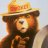# Convert TKP Relative Movement indicator to ToS

S

#### sanri

##### New member
Hello,

Code:
``````study("TKP True Relative Movement", shorttitle="TKP-TRM ", overlay=true, format=format.price, precision=4)
Note = input( 0, title = "TSI standard values are 25, 13, 13, and RSI is 14. Can change the default values to these for 'Slow TRM'")
long = input(title="TSI-Long Length", type=input.integer, defval=25)
short = input(title="TSI-Short Length", type=input.integer, defval=5)
signal = input(title="TSI-Signal Length", type=input.integer, defval=14)
price = close
double_smooth(src, long, short) =>
fist_smooth = ema(src, long)
ema(fist_smooth, short)
pc = change(price)
double_smoothed_pc = double_smooth(pc, long, short)
double_smoothed_abs_pc = double_smooth(abs(pc), long, short)
tsi_value = 100 * (double_smoothed_pc / double_smoothed_abs_pc)
TSI_Signal_Line = (ema(tsi_value, signal))

////RSI

src = close, len = input(5, minval=1, title="RSILength")
up = rma(max(change(src), 0), len)
down = rma(-min(change(src), 0), len)
rsi = down == 0 ? 100 : up == 0 ? 0 : 100 - (100 / (1 + up / down))
rsiBuyfilterlevel = input(50, minval = 1, title = "RSI cross above Buy Level")
rsiSellfilterlevel = input(50, minval = 1, title = "RSI cross below Sell Level")

///Bar Coloring

TSI_Sell = ( (tsi_value < TSI_Signal_Line) and (rsi <rsiSellfilterlevel))How do I convert this ATR% chart study into a scanner watchlist column? Questions 5